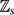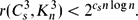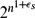Home

# Improved Bounds for the Ramsey Number of Tight Cycles Versus Cliques

## Abstract

The 3-uniform tight cycle Cs 3 has vertex set${\mathbb Z}_s$ and edge set {{i, i + 1, i + 2}: i${\mathbb Z}_s$ }. We prove that for every s ≢ 0 (mod 3) with s ⩾ 16 or s ∈ {8, 11, 14} there is a cs > 0 such that the 3-uniform hypergraph Ramsey number r(Cs 3, Kn 3) satisfies$$\begin{equation*} r(C_s^3, K_n^3)< 2^{c_s n \log n}.\ \end{equation*}$$
This answers in a strong form a question of the author and Rödl, who asked for an upper bound of the form$2^{n^{1+\epsilon_s}}$ for each fixed s ⩾ 4, where εs → 0 as s → ∞ and n is sufficiently large. The result is nearly tight as the lower bound is known to be exponential in n.

Hide All

# Improved Bounds for the Ramsey Number of Tight Cycles Versus Cliques

## Metrics

### Full text viewsFull text views reflects the number of PDF downloads, PDFs sent to Google Drive, Dropbox and Kindle and HTML full text views.

Total number of HTML views: 0
Total number of PDF views: 0 *Loading metrics...

### Abstract viewsAbstract views reflect the number of visits to the article landing page.

Total abstract views: 0 *Loading metrics...

* Views captured on Cambridge Core between <date>. This data will be updated every 24 hours.

Usage data cannot currently be displayed.